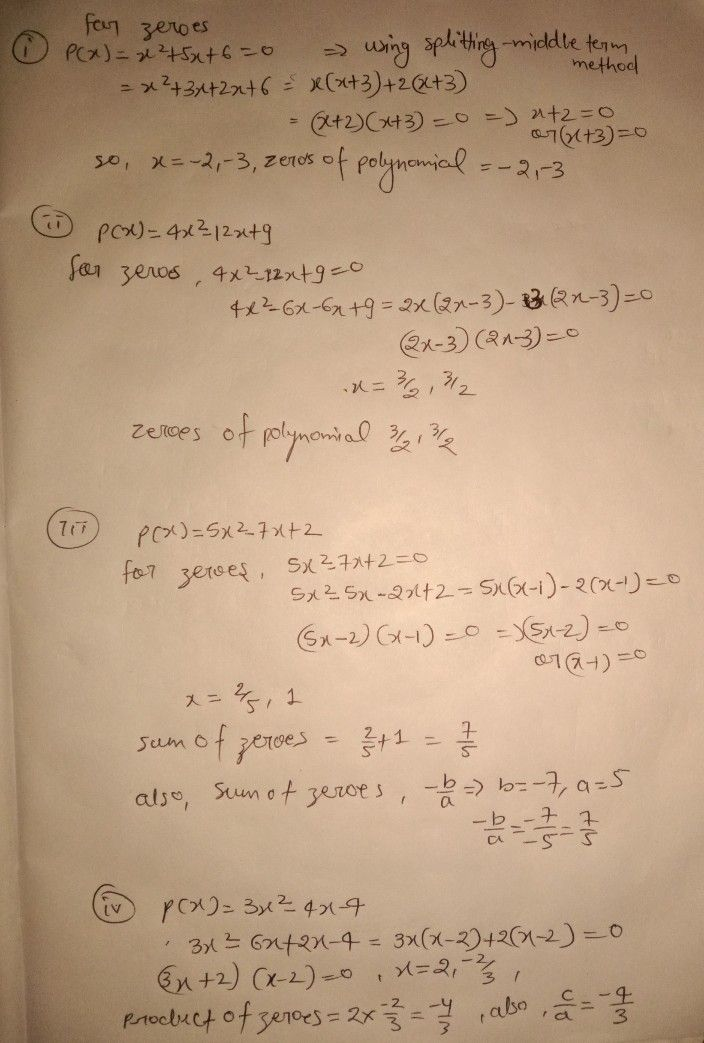Symbol
ProblemFind the zeroes of the polynomial p(x) $x^{2}+3x+6$ Find the zeroes the polynomial p(x) $=4x^{2}-12x+9$ 3. Find the sum of the zeroes of the polynomial $2\left(x\right)=5x^{2}-7x+2$ 4. Find the product of the zeroes of the polynomial $p\left(x\right)=3x^{2}-4x-4$
10th-13th grade
Calculus
Search count: 112
SolutionQanda teacher - pradeepkum